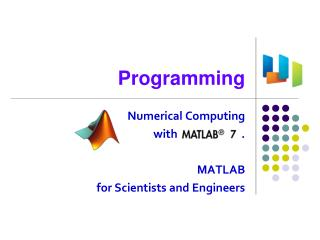Download PresentationProgramming# Programming - PowerPoint PPT Presentation

Download Presentation##### Programming

Download Policy: Content on the Website is provided to you AS IS for your information and personal use and may not be sold / licensed / shared on other websites without getting consent from its author. While downloading, if for some reason you are not able to download a presentation, the publisher may have deleted the file from their server.

- - - - - - - - - - - - - - - - - - - - - - - - - - - E N D - - - - - - - - - - - - - - - - - - - - - - - - - - -
##### Presentation Transcript

1. Programming Numerical Computing with . MATLAB for Scientists and Engineers

2. You will be able to • Write simple MATLAB programs using if statement, • Write simple MATLAB programs using for statement, • Convert a for-loop based program into a matrix operation version.

3. Relational Operators • Operators • Results are logicals < <= > >= == ~= a = 5 < 8 b = [1:2:8] > 3 c = [2:2:13] == [ 0:3:17 ] A = randint(3,4,10) A>5 A(A>5) = 5

4. Exercise 1: Hate 4 • Generate a 5x6 matrix A with random integers between 0 and 10. • If some elements of matrix A is 4, replace it with 0. • If some elements of matrix A is odd, subtract 1 from the elements. hate4.m

5. Logical Operators • Operators • Built-in Functions • Examples & | ~ and(A,B) or(A,B) not(A) xor(A,B) all(A) any(A) find(A) find(A>d)

6. Conditional Statement: if • if condition • if condition – else F n = input('Enter an integer: '); if mod(n,2) fprintf('%d is an odd integer.\n', n ); end x<0 T S1 S1 S2 n = input('Enter an integer: '); if mod(n,2) fprintf('%d is an odd integer.\n', n ); else fprintf('%d is an even integer.\n', n ); end F x<0 T S1 S1 S2

7. Exercise 2 Volume in a Water Tank • Find the volume of the water tank below when the depth is h. 10m 8m 8m 8m h m 6m 6m

8. Solution 2 • Function m-file • Commands water_volume.m

9. if – elseif – else – end • Nested If if A x = a else if B x = b else if C x = c else x = d end end end if A x = a elseif B x = b elseifC x = c else x = d end F F F if elseif elseif T T T S1 S2 S3 S4

10. For Loops for n=a:b statements end i=0; for t=0:0.1:100 i = i+1; x(i) = sin(2*pi*200*t); end for n=1:5 for m=5:-1:1 a(n,m)=n^2 + m^2; end end t=0:0.1:100; x = sin(2*pi*200*t); n=1:5; m=1:5; [nn,mm]=meshgrid(n,m); a=nn.^2 + mm.^2;

11. Example • Taylor series of sine function • Write a function y= Tsin(x,n), which is the partial sum of n-terms.

12. Exercise 3 • Taylor series of exp(x). • Write a function y= Texp(x,n), which is the partial sum of n-terms. Use Texp() to plot exp(x), Texp(x,5), Texp(x,10), Texp(x,100).

13. Solution 3 • Function m-file • Commands and Screenshot Texp.m

14. While Loops i=0;t=0; while t<=100 i = i+1; x(i) = sin(2*pi*200*t); t = t+0.1; end i=0; for t=0:0.1:100 i = i+1; x(i) = sin(2*pi*200*t); end t=0:0.1:100; x = sin(2*pi*200*t);

15. Lotto Numbers • disp('I generate Lotto numbers'); • disp('Good Luck!'); • B=[]; • for i=1:5 • L=0; • while L ~= 6, • fprintf('Try - %d \n ', i ); • A=floor(rand(1,6)*45)+1; • A=unique(A); • L=length(A); • end • B(i,:) = A; • pause(1); • end • B

16. Branch apple=100; if apple < 5 disp('few'); elseif apple < 20 disp('a few'); else disp('a lot'); end mon = 10; switch mon case 1 month = 'January'; case 2 month = 'February'; otherwise month = 'Others'; end

17. Branch x = 2.7; units = 'm'; switch units case {'inch', 'in'} y = x * 2.54; case {'feet', 'ft'} y = x * 2.54 * 12; case {'meter', 'm'} y = x * 100; case {'milimeter', 'mm'} y = x / 10; case {'centimeter', 'cm'} y = x; otherwise y = nan; end Unlike C, no break!